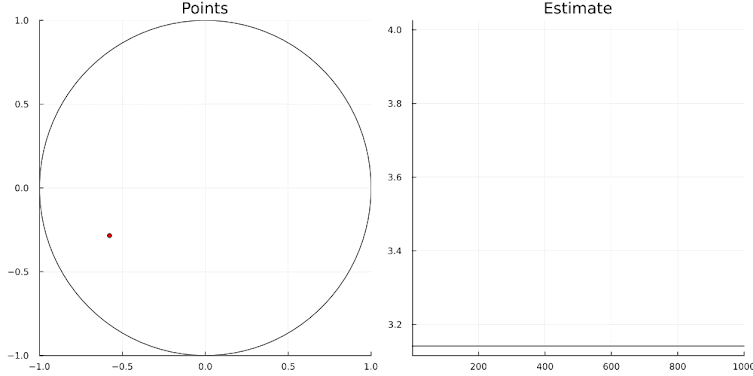## Wednesday, September 20, 2023

### Julia vs Python

I gave a talk to economists (i.e., not professional programmers) about a Julia project we were working on. Julia is famous for its speed. It uses LLVM  as back-end for its JIT (Just In Time) compilation. As seeing is believing, here is an example algorithm which is used to compare performance between different languages and tools. This example was chosen as it is small, easy to explain, and easy to program while still showing meaningful time differences.

We have a square $$[-1,+1]\times[-1,+1]$$ and an inscribing circle with radius $$1$$. See the dynamic figure below. Their areas are $$4$$ and $$\pi$$ respectively. The idea is to draw $$n$$ points \begin{align}& x_i \sim U(-1,+1) \\ & y_i \sim U(-1,+1)\end{align}Let $$m$$ be the number of points inside the circle, i.e. with $x_i^2+y_i^2\lt 1$ Obviously, from the ratio of the areas, we have $\frac{m}{n} \approx \frac{\pi}{4}$ It follows that an estimate of $$\pi$$ is $\hat{\pi}=4\frac{m}{n}$Simulation with n=1000

Here, I look at five different implementations of this simulation, in increasing speed:
1. A straightforward implementation in Python using a for loop. This is very slow.
2. A GAMS version of this algorithm. Just for the heck of it.
3. A set-driven GAMS version replaces the inner loop with a sum. This version was suggested by .
4. A Python version that uses the Numba JIT compiler . Numba uses the same LLVM  back-end as Julia. This is 10 times as fast. Numba does not support f-strings, so the print statement must be changed. This version was not in my talk. I added it for completeness. And out of curiosity.
5. A Python implementation using NumPy . Here, we use arrays instead of explicit loops. Of course, NumPy will still use loops behind the scenes, but that is done inside a more high-performance C library implementation. This formulation gives a big performance boost. Lesson: in Python, loops are very expensive. This variant is not very close to our original version. It is, however, significantly faster than the Numba version.
6. This is the same intuitive implementation as in 1., but now using Julia. This is the fastest of all, by a large margin. The looped version is very fast and it will require some esoteric code to improve on this. 
A single simulation uses 1 million points. We repeat this 10 times.

#### 1. Python loop

Output:

This is a scalar loop executed in pure Python. It is the slowest version.

#### 2. GAMS scalar loop

Output:

A bit faster than the Python loop.

#### 3. Set-driven GAMS formulation

Output:

This is twice as fast as the "for i" loop in the previous GAMS fragment.

#### 4. Python/Numba loop

Output:

Here we use the Numba JIT compiler to speed up the loop. We achieve a speed-up of about 10x. Nothing to sneeze at.

#### 5. Python/NumPy without loops

Output:

Here we use NumPy arrays so we don't need loops. This is faster than the Numba example. This gives us a 25x speed-up.

#### 6. Julia loop

Output:

Note the use of $$\in$$ and $$4m$$. This Julia version is very fast. We recorded here a 150x speed-up compared to the first example. The main expenditure here is the random number generation.

The performance is summarized in the following table:

ImplementationTime for 10
simulations (seconds)
Approximate
speed-up
1. Pure Python with loops4.66
2. GAMS scalar loop2.272x
3. Set-driven GAMS1.174x
4. Python/Numba with loops0.45 10x
5. Python/NumPy without loops0.1825x
6. Julia with loops0.03150x

I find these numbers to be rather astounding.

It is a bit of a surprise to me that GAMS is about 2x as fast as Python, using the same loop-based algorithm. As GAMS is not a general-purpose programming language, including it here is a bit of a gimmick. When programming in Python, it is not unusual to write several different implementations: one that works and then some faster versions. It certainly is beneficial in this case. An argument for using Julia could be that our first, intuitive, implementation is already very fast.

### Conclusion

Explicit loops in Python are expensive. We have to rewrite things considerably to get decent performance. This is not the case for Julia: loops are cheap. A first, straightforward implementation in Julia performs better than our Python attempts.

All versions here are serial. Sometimes, NumPy may do some parallelization under the hood.

### References

1. The LLVM Compiler Infrastructure, https://llvm.org/
2. https://numba.pydata.org/
3. https://numpy.org/
4. Thomas Rutherford, University of Wisconsin, Private Communication
5. https://julialang.slack.com/archives/C67910KEH/p1696273686727619?thread_ts=1696266934.797769&cid=C67910KEH demonstrates some Julia code using VectorizedRNG.

1.Hello, how did you create the animated graphs?

1.@gif macro in plots.jl

2.You're testing the speed of random number generation.

1.No. (1) cpython's random() is written in C. It is not dumb code. (2) The difference in timings can be reproduced by doing other things than generating random numbers. (3) This behavior has been shown by other benchmarks.